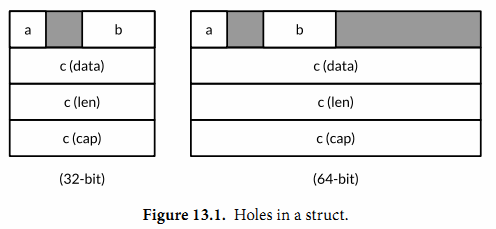## 13.1. unsafe.Sizeof, Alignof 和 Offsetof

unsafe.Sizeof函数返回操作数在内存中的字节大小，参数可以是任意类型的表达式，但是它并不会对表达式进行求值。一个Sizeof函数调用是一个对应uintptr类型的常量表达式，因此返回的结果可以用作数组类型的长度大小，或者用作计算其他的常量。

import "unsafe"
fmt.Println(unsafe.Sizeof(float64(0))) // "8"


Sizeof函数返回的大小只包括数据结构中固定的部分，例如字符串对应结构体中的指针和字符串长度部分，但是并不包含指针指向的字符串的内容。Go语言中非聚合类型通常有一个固定的大小，尽管在不同工具链下生成的实际大小可能会有所不同。考虑到可移植性，引用类型或包含引用类型的大小在32位平台上是4个字节，在64位平台上是8个字节。

bool 1个字节
intN, uintN, floatN, complexN N/8个字节(例如float64是8个字节)
int, uint, uintptr 1个机器字
*T 1个机器字
string 2个机器字(data,len)
[]T 3个机器字(data,len,cap)
map 1个机器字
func 1个机器字
chan 1个机器字
interface 2个机器字(type,value)

Go语言的规范并没有要求一个字段的声明顺序和内存中的顺序是一致的，所以理论上一个编译器可以随意地重新排列每个字段的内存位置，虽然在写作本书的时候编译器还没有这么做。下面的三个结构体虽然有着相同的字段，但是第一种写法比另外的两个需要多50%的内存。

                               // 64-bit  32-bit
struct{ bool; float64; int16 } // 3 words 4words
struct{ float64; int16; bool } // 2 words 3words
struct{ bool; int16; float64 } // 2 words 3words


unsafe.Alignof 函数返回对应参数的类型需要对齐的倍数. 和 Sizeof 类似, Alignof 也是返回一个常量表达式, 对应一个常量. 通常情况下布尔和数字类型需要对齐到它们本身的大小(最多8个字节), 其它的类型对齐到机器字大小.

unsafe.Offsetof 函数的参数必须是一个字段 x.f, 然后返回 f 字段相对于 x 起始地址的偏移量, 包括可能的空洞.

var x struct {
a bool
b int16
c []int
}32位系统：

Sizeof(x)   = 16  Alignof(x)   = 4
Sizeof(x.a) = 1   Alignof(x.a) = 1 Offsetof(x.a) = 0
Sizeof(x.b) = 2   Alignof(x.b) = 2 Offsetof(x.b) = 2
Sizeof(x.c) = 12  Alignof(x.c) = 4 Offsetof(x.c) = 4


64位系统：

Sizeof(x)   = 32  Alignof(x)   = 8
Sizeof(x.a) = 1   Alignof(x.a) = 1 Offsetof(x.a) = 0
Sizeof(x.b) = 2   Alignof(x.b) = 2 Offsetof(x.b) = 2
Sizeof(x.c) = 24  Alignof(x.c) = 8 Offsetof(x.c) = 8